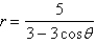# [Solved] Consider the Polar Equation (A)find the Eccentricity and an Equation

Question 9
Essay

## Consider the polar equation(a)Find the eccentricity and an equation of the directrix of the conic. (b)Identify the conic. (c)Sketch the curve.

10+ million students use Quizplus to study and prepare for their homework, quizzes and exams through 20m+ questions in 300k quizzes.

### Psychology

Explore our library and get Personality Psychology Homework Help with various study sets and a huge amount of quizzes and questions

821

Study sets

15K

Quizzes

1.4M

Questions

Upload material to get free accessInvite a friend and get free accessSubscribe and get an instant access